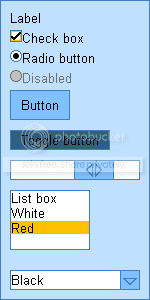-=+=- -=+=- -=+=- -=+=- -=+=- -=+=- -=+=- -=+=- -=+=- -=+=- -=+=- -=+=- -=+=- -=+=- -=+=- -=+=- -=+=- -=+=- -=+=- -=+=- -=+=- -=+=- -=+=- -=+=- -=+=- -=+=- -=+=- -=+=- -=+=- -=+=- (c) WidthPadding Industries 1987 0|151|0 -=+=- -=+=- -=+=- -=+=- -=+=- -=+=- -=+=- -=+=- -=+=- -=+=- -=+=- -=+=- -=+=- -=+=- -=+=- -=+=- -=+=- -=+=- -=+=- -=+=- -=+=- -=+=- -=+=- -=+=- -=+=- -=+=- -=+=- -=+=- -=+=- -=+=- SoCoder -> Showcase Home -> Functions Libraries Featuring useful single functions or whole libraries to give your language new abilities. Older -->JONK Event-driven JSON parser module for BlitzMax Cower Blitz Max Cross PlatformBakefont3 HoboBen , Python, CPHP Error Better error reporting in PHP JL235 Flash Cross PlatformSVG Tech Demo 2 While only an early release, full source code is provided including the XML Parser for loading SVG files. HoboBen , Cobra WindowsNUI Toolkit NUIT is a skinnable 2D user interface toolkit for BlitzMax that uses Max2D for rendering Cower Blitz MaxBufferedGLMax2D An OpenGL Max2D module that attempts to minimize state changes and uses retained-mode rendering (unlike GLMax2D's use of immediate-mode rendering). Cower Blitz Max Cross PlatformJay's BMax Framework Scream as you look into my code! Jayenkai Blitz Max Cross PlatformOpalescence A small GUI toolkit for Cobra flying_cucco CobraLuGI LuGI is a set of modules for providing an interop layer between BlitzMax and Lua as well as wrapping BlitzMax types for use within Lua. Cower Blitz MaxInstance DSL a small domain specific langauge for setting up new instances of instances of a class JL235 Ruby Browser BasedShortcut Reader/Writer for BlitzBasic and Cobra For Cobra (+Blitz!): custom icons, comment/tooltips, shortcuts with command line arguments, it's all in there! HoboBen , Cobra WindowsMersenne Twister Random Number Userlib Use Mersenne Twister to generate random numbers. Evil Roy Ferguson Blitz WindowsBlitz 2D in 3D (v2) 2D sprites in 3D. Jayenkai Blitz WindowsLlama Simple 2D Java Game Library JL235 Ruby Server Based Older -->